# Types of Visualization in Python

Dr McFadden has 20+ years of experience in IT and over 12 years in teaching college courses across multiple colleges, both in person and online.

This lesson provides an overview of Python's GraphWin objects and methods that are used in graphical processing. This includes plot, location, shape, size, and color.

## GraphWin

GraphWin is a class in GNU open-source software (graphics.py). It was written by John Zelle to be used with a textbook on Python. It simplifies writing graphics code in Python so that it is easier to learn. GraphWin uses the standard Python graphics package Tkinter, which is usually bundled with Python interpreter. To use in your program you need to download and import it.

## Graphics Window

To create a Graphics Window, you create an instance of GraphWin, passing it the title of the window, width, and height. For example:

`from graphics import *def main():    win = GraphWin(''Learn Graphics'', 250, 250) # specifies graphics window size 250x250    win.getMouse() # pause for click in window to close    win.close()main()`

In this code we specify the title to be 'Learn Graphics' and the window size 250 by 250. When we run it, we get a pop-up window as shown in the Figure 1 below. Clicking on the window will close it.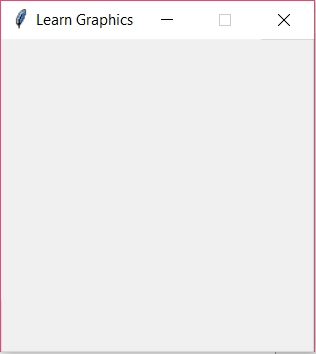## Plot

To draw a single pixel we can use the plot method which takes as parameters an x coordinate, y coordinate, and a string for color. The color parameter is optional and the default color is black.

For example, to draw a red pixel in the middle of the graphics window in above code, we add:

win.plot(125, 125, "red")

When we run it, we get a pop-up window as shown in the Figure 2 below. The pixel may be hard to see so we circled it with red pen in the image.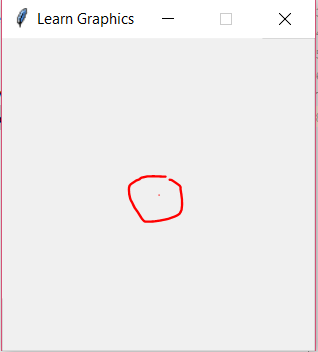## Location

As you have seen in the plot example above, we use the x and y coordinates to specify the location of the object within the graphics window. Looking at the graphics window, the top left corner is location x=0 and y=0. Then going across horizontally, the values of x coordinate grow. For our graphics window we have x values from 0 to 250. Similarly for y coordinate, as we go down vertically, the values grow and for our graphics window they are again 0 to 250.

## Shapes

GraphWin supports a number of graphics objects such as Point, Circle, Rectangle, Oval, and Polygon. For each of these we specify coordinates that determine the location and the size of the object. In some cases we need additional parameter such as the radius for the circle.

### Point

The Point object creates a point at x and y coordinate. So for example to create a Point in the middle of our graphics window, we need:

Point(125,125)

Once we create the object, we then need to specify that it be drawn in the graphics window. We use the object's draw method and specify our graphics window instance as parameter:

g = Point(125,125)
g.draw(win)

The output will look identical to the plot example except the dot will be black as the default color.

Point object also has getX and getY methods to return its location.

### Circle

The Circle object has two parameters: Point of its center location and the radius value. It also has getCenter and getRadius methods to return those values back.

For example we can place the circle in the middle again and give it the radius 100:

g = Circle(Point(125,125), 100)

When we run the code, it will look like the Figure 3 below: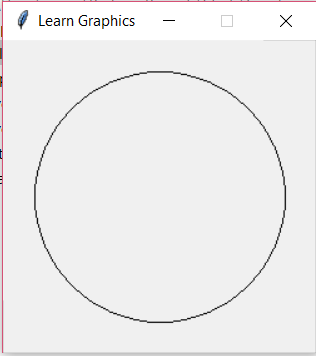### Rectangle

The Rectangle object also has two parameters but they are both a Point object. The Point values determine the location of the opposite corners of the rectangle. It also has getCenter method that returns the value of the point in the center of the Rectangle object.

Here is an example:

g = Rectangle(Point(100,100), Point(150, 150))

And what it looks like in the Figure 4 below: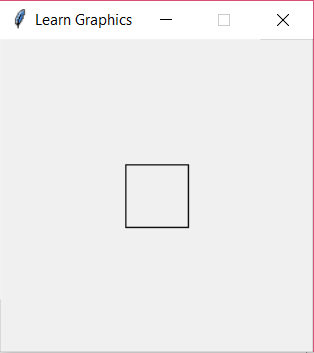### Oval

The Oval object works the same way as the Rectangle with two Point objects as parameters and getCenter method. But instead of creating a rectangle it creates the oval within the boundaries of the two Point values.

For example:

g = Oval(Point(100,100), Point(200, 150))

And what it looks like in the Figure 5 below: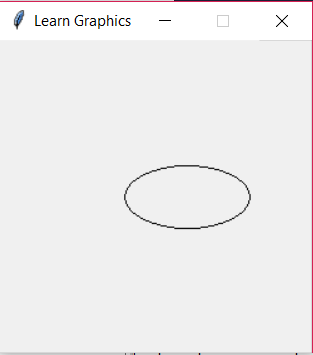### Polygon

The Polygon object has variable number of Point parameters depending on the polygon that needs to be formed. Each Point is a vertex of the polygon. It also has getPoints method which returns the list of the points in the Polygon object.

For example:

To unlock this lesson you must be a Study.com Member.

### Register to view this lesson

Are you a student or a teacher?

### Unlock Your Education

#### See for yourself why 30 million people use Study.com

##### Become a Study.com member and start learning now.
Back
What teachers are saying about Study.com

### Earning College Credit

Did you know… We have over 160 college courses that prepare you to earn credit by exam that is accepted by over 1,500 colleges and universities. You can test out of the first two years of college and save thousands off your degree. Anyone can earn credit-by-exam regardless of age or education level.